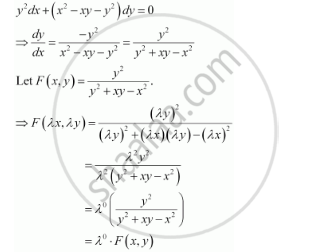Share

# Which of the Following is a Homogeneous Differential Equation? - CBSE (Science) Class 12 - Mathematics

ConceptMethods of Solving First Order, First Degree Differential Equations Homogeneous Differential Equations

#### Question

Which of the following is a homogeneous differential equation?

(A) (4x + 6y + 5) dy – (3y + 2x + 4) dx = 0

(B) (xy) dx – (x3 + y3) dy = 0

(C) (x3 + 2y2) dx + 2xy dy = 0

(D) y2 dx + (x2 – xy – y2) dy = 0

#### Solution

Function F(xy) is said to be the homogenous function of degree n, if

F(λx, λy) = λn F(xy) for any non-zero constant (λ).

Consider the equation given in alternativeD:Hence, the differential equation given in alternative D is a homogenous equation.

Is there an error in this question or solution?

#### APPEARS IN

NCERT Solution for Mathematics Textbook for Class 12 (2018 to Current)
Chapter 9: Differential Equations
Q: 17 | Page no. 407
Solution Which of the Following is a Homogeneous Differential Equation? Concept: Methods of Solving First Order, First Degree Differential Equations - Homogeneous Differential Equations.
S